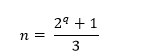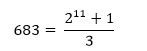# Check whether the given number is Wagstaff prime or not in Python

PythonServer Side ProgrammingProgramming

Suppose we have a number n. We have to check whether n is Wagstaff prime or not. As we know Wagstaff prime is a prime number which is in the following form.where q is an odd prime number.

So, if the input is like n = 683, then the output will be True n can be represented asSo here q = 11. And q is odd prime.

To solve this, we will follow these steps −

• if num is prime and (num*3 - 1) is also prime, then
• return True
• return False

Let us see the following implementation to get better understanding −

## Example Code

Live Demo

def isPrime(num):
if num > 1:
for i in range(2, num):
if num % i == 0:
return False
return True
return False

def power_of_two(num):
return num and not(num & (num - 1))

def solve(num) :
if isPrime(num) and power_of_two(num * 3-1):
return True
return False

n = 683
print(solve(n))

## Input

683


## Output

True